# KSEEB Solutions for Class 6 Maths Chapter 4 Basic Geometrical Ideas Ex 4.3

Students can Download Chapter 4 Basic Geometrical Ideas Ex 4.3 Questions and Answers, Notes Pdf, KSEEB Solutions for Class 6 Maths helps you to revise the complete Karnataka State Board Syllabus and score more marks in your examinations.

## Karnataka State Syllabus Class 6 Maths Chapter 4 Basic Geometrical Ideas Ex 4.3

Question 1.
Name the angle in the given figure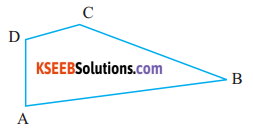Solution:

Question 2.
In the given diagram, name the point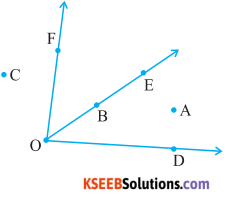Solution:
a) In the interior of ∠DOE
A

b) In the exterior of ∠EOF
C.A.D

c) On ∠EOF
B.E.D.FQuestion 3.
Draw rough diagrams of two angles such that they have
Solution:
a) One point in common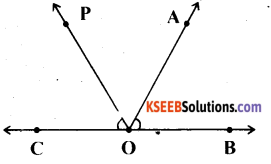∠COD and Z AOD have point O m common
Two Points in common

b) Two points in common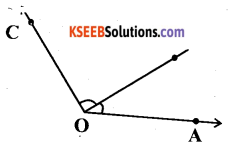∠AOB and ∠BOC have point O and B in common

c) Three points in common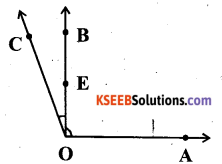∠AOB and ∠BOC have points O, E, B in common

d) Four points in common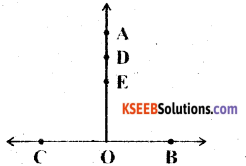∠BOA and ∠COA have points O, E, D, A in commone) One ray in common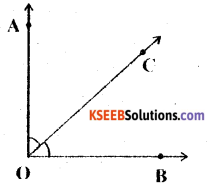Ray $$\overrightarrow{OC}\$$ is common between ∠BOC and ∠AOC.